###### eISSN: 2577-8242# Fluid Mechanics Research International JournalReview Article Volume 2 Issue 4

# CFD simulation of the moonpool on the total resistance of a drillship

#### Sivabalan Ponnappan,1 Surendran Sankunny2 function clickButton(){ var name=document.getElementById('name').value; var descr=document.getElementById('descr').value; var unCopyslNo=document.getElementById('unCopyslNo').value; document.getElementById("mydiv").style.display = "none"; $.ajax({ type:"post", url:"https://medcraveonline.com/captchaCode/server_action", data: { 'name' :name, 'descr' :descr, 'unCopyslNo': unCopyslNo }, cache:false, success: function (html) { //alert('Data Send');$('#msg').html(html); } }); return false; } Verify Captcha Regret for the inconvenience: we are taking measures to prevent fraudulent form submissions by extractors and page crawlers. Please type the correct Captcha word to see email ID.function refreshPage(){ $("#mydiv").load(location.href + " #mydiv"); }$(document).ready(function () { //Disable cut copy paste $('#msg').bind('cut copy paste', function (e) { e.preventDefault(); }); //Disable mouse right click$("#msg").on("contextmenu",function(e){ return false; }); }); .noselect { -webkit-touch-callout: none; /* iOS Safari */ -webkit-user-select: none; /* Safari */ -khtml-user-select: none; /* Konqueror HTML */ -moz-user-select: none; /* Firefox */ -ms-user-select: none; /* Internet Explorer/Edge */ user-select: none; /* Non-prefixed version, currently supported by Chrome and Opera */ cursor: none; }

1Research Scholar, Department of Ocean Engineering, Indian Institute of Technology Madras, India
2Professor, Department of Ocean Engineering, Indian Institute of Technology Madras, India

Correspondence: Surendran Sankunny, Professor, Department of Ocean Engineering, Indian Institute of Technology Madras, Chennai, India, Tel 91-044 2257 4815,

Received: June 14, 2018 | Published: August 16, 2018

Citation: Ponnappan S, Sankunny S. CFD simulation of the moonpool on the total resistance of a drillship. Fluid Mech Res Int. 2018;2(4):164-169. DOI: 10.15406/fmrij.2018.02.00034

# Abstract

Moonpools are openings right through the hull from continuous deck to bottom of the ship, allowing equipment or mini-submarines to be put into the water at a location on the vessel with minimum ship motion. Open moonpools in a drillship are causing additional resistance when the ship is in forward speed. It was shown that the water inside the moonpool started to oscillate at forward speed. This water particle motion inside the moonpool is mainly depended on the geometry, moonpool depth, and encountered wave frequency. Out of this, moonpool geometry is a one of the key factor for the performance of the moonpool. The varying cross-section geometry is one of the practically possible and economically feasible solution to reduce the oscillation to a considerable level is attempted in this paper. Also the resistance caused by the moonpool and the free surface generated around the hull are investigated with the use of computer simulation.

Keywords: Moonpool, Free surface elevation, water motion.

# Introduction

A moonpool is meant for access to underwater part from onboard ship. It is located mostly at the midship region of the drillship for under water operations either in square or rectangular in shape. In drillship, these openings are used for supply the raiser and other drilling equipment through it. This drillship moonpool is mainly subjected to two types of motions of water mass inside the column namely, piston mode and sloshing mode. The piston mode is a type of water particle motion where the water particles can be seen as a “plug” which oscillates in the vertical direction. In sloshing mode the movement of water plugs in the horizontal direction and makes a standing wave effect on the side wall of the moonpool. This piston and sloshing mode play a major role in vessel’s surge and heave motion while the drill ship in operation/transit and its lead to a larger quantity of green water on the weather deck. In the case of rectangular moonpool the dominating mode is sloshing where as in square moonpool the piston mode of oscillation is the dominant one. Because of this water motion inside the moonpool the drillship regular activities may be affected. Also this water motion is the reason for a large quantity of sea water can enter on to the continuous deck of the vessel. If this quantity of water added with the green water at the deck because of the waves then the net effect may be cause critical situation of the vessel. Also this water entry leads to the down time period of the drillship, because the crew members working on the drillship feel instability over the deck. Therefore this entry of water to the deck should be minimized to better performance of the drillship.

This water motion inside the moonpool also increases the drillship resistance, when the ship is in transit condition. The moonpool opening can be close during the transit by hatch cover, but any failure happened in the operation of the hatch cover leads to stop the drillship operation. This may cause major economic loss to the company, therefore most of the transit the drillship hatch cover in open condition.

In regular moonpool geometry the water motion inside the moonpool when the drillship in operation mode is a considerable level, it further increases when the ship is in transit condition. This leads to number of studies in changing the moonpool geometry. Some of the studies are discussed here.

Aalbers1 paid attention on moonpool hydrodynamics and formulated the mathematical model for relative water motion inside the moonpool. In both the numerical and experimental study the influence of damping mechanism was also evaluated. Ahmed et al.,2 numerically simulated the free surface flow around the VLCC hull form with the use of potential flow code and viscous flow finite volume code and compared the same with the available results. The geometry of the moonpool is the one of the important parameter in the case of formation of vortices. Fukuda3 conducted research on the behavior of the water column oscillations in different shapes of moonpool and its effects on the vessel motion .The author observed that the flow inside the moonpool mainly depended on the shape of the moonpool. The flow inside the moonpool is like turbulent in nature, also the aft end of the drillship. To simulate the free surface around the hull a suitable model considered as Explicit Algebraic Stress Model (EASM) by Gatski et al.4 The water motion inside the moonpool will cause the resonance when it is in a critical level. Gaillarde et al.,5 carried out experiments in moonpool with different motion minimizing attachments to reduce the free surface elevation inside the moonpool. The authors also attempted with varying cross section of moonpool along the depth of the ship to reduce the water motions.

The experimental and numerical study of resistance and flow field was carried out by Guo et al.,6 in KVLCC2 hull. The EASM and SST turbulence model were used in numerical study. The results of both the model have good agreement with the experimental study. Also the detected outputs from turbulence models in resistance, sinkage, trim and the free surface are found to be good. The overall resistance of ship can be divided in to two as wave resistance and viscous resistance.7,8 Out of these two resistance part, the drillship with moonpool does not affect the wave resistance, but it affect the viscous pressure resistance due to pressure imbalance generated by the moonpool. Kim et al.,9 studied the stability of Rankine Panel method used in prediction of ship resistance. Molin10 derived theoretical formulas for both the piston mode and sloshing mode natural frequency of moonpool in two dimensional and three dimensional cases using linearized potential flow theory. In this study the author formulated the free surface shape for both the piston and sloshing mode.

Veer et al.,11 performed model test on moonpool of the drill ship with different length to breadth ratio and studied the correlation between the shape of moonpool and increase of resistance. The authors arrived at the variation of free surface elevation inside moonpool according to the ratio of draft to moonpool breadth. The present study mainly on the changing the moonpool geometry by introducing cut-out angles in moonpool and the performance is compared with the zero cut-out angle moonpool. The cut-out moonpools are otherwise called as the varying cross-section moonpool, because its area gradually decreases from bottom to top. This decrement decreases the water motion inside the moonpool to a considerable level both in operation and transit mode of the drillship. The performance of the cut-out moonpools are comparatively good than the zero cut-out angle moonpool. In this study two different cut-out moonpools (20 degree and 30 degree) are used for the computer simulations for the study of free surface and the resistance.

# Moonpool modelling

The computer simulation is done by the use of SHIPFLOW package for different Froude numbers and different draft of the drillship. The total resistance coefficient, free surface around the hull, trim and sinkage are found from the computer simulation. The body plan of the drillship and the main particulars of drillship are given in Figure 1 and Table 1 respectively. A full length drillship is used for the computer simulation.

 Specification       (unit) Value Length (LOA)         (m) 292 Length(LBP)           (m) 280 Breadth                 (m) 50 Draft                      (m) 16.5 Depth                     (m) 25.48 Displacement       (ton) 1,94,156

Table 1 Principal dimensions of ship

All the iteration the same hull is used with different moonpool configurations. All the rectangular, 20 degree cut-out moonpool and 30 degree cut-out moonpool are placed at the midship area of the hull. Clear views of cut-out moonpools and rectangular moonpool are given in Figure 2.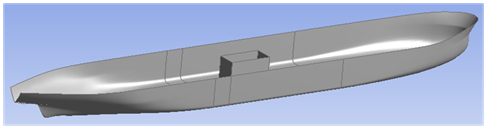Figure 2(A) Drillship hull with rectangular moonpool.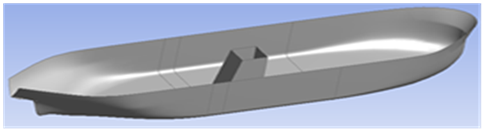Figure 2(B) Drillship hull with 20 degree cut-out moonpool.Figure 2(C) Drillship hull with 30 degree cut-out moonpool.

In cut-out moonpool case the cut-out is given in the aft end of the moonpool and the other end (forward end) is simply a vertical wall. This cut-out angle gradually decreases the cross-sectional area of the moonpool from bottom to top and the moonpool like a varying cross-section moonpool.13

Computer simulation
The aim of Computational Fluid Dynamics (CFD) is to solve the basic equations which govern the flow: continuity equation and the Navier-Stokes equations.

Continuity Equation
$\frac{\partial \rho }{\partial t}+\nabla .\left(\rho U\right)=0$                                                                                 (1)
where ρ is fluid density, t is time and U is the velocity vector.
For the case of steady state flow, Eq. (1) is reduced to
$\nabla .\left(\rho U\right)=0$                                                                                           (2)

As all the fluids is incompressible, the equation (2) becomes
$\frac{\partial u}{\partial x}+\frac{\partial v}{\partial y}+\frac{\partial w}{\partial z}=0$                                                                                 (3)

Navier-Stokes equations
The Navier-Stokes equation for an incompressible Newtonian fluid can now be written as
$\rho \frac{DU}{Dt}=-\nabla p+\mu {\nabla }^{2}U+\rho g\text{\hspace{0.17em}}\text{\hspace{0.17em}}\text{\hspace{0.17em}}$                                                               (4)
Where $\frac{DU}{Dt}=$ material derivative $g=$ Gravity

Shipflow theory
In the present study SHIPFLOW package is used for the computational study. The features of the SHIPFLOW CFD code has discussed here. In this the solver solves the flow problem in zonal approach. In overall view it divides the object in to three zones with three different solution approaches. The zone 1 uses the potential flow solver with Rankine source panel method. In the potential flow method the SHIPFLOW code can be run in both linear and non-linear mode. It solves the forward portion of the ship hull.
${\nabla }^{2}\varphi =\frac{{\partial }^{2}\varphi }{\partial {x}^{2}}+\frac{{\partial }^{2}\varphi }{\partial {y}^{2}}+\frac{{\partial }^{2}\varphi }{\partial {z}^{2}}=0$                                                (5)

In Zone 2 in SHIPFLOW the boundary layer is computed using a momentum-integral method. It is based on streamlines, which are automatically traced from the potential-flow solution.

In Zone 3 the governing equations are the Reynolds-Averaged Navier-Stokes (RANS) equations, obtained by averaging the time dependent Navier-Stokes equations over the entire length and time scales of the turbulent fluctuations. It solves the aft end of the ship hull by turbulent flow solver
$\frac{\partial }{\partial t}\left(\rho \right)+\frac{\partial }{\partial {x}_{i}}\left(\rho {u}_{i}\right)=0$                                                         (6)
$\begin{array}{l}\frac{\partial }{\partial t}\left(\rho {u}_{i}\right)+\frac{\partial }{\partial {x}_{i}}\left(\rho {u}_{i}{u}_{j}\right)=-\frac{\partial p}{\partial {x}_{i}}+\frac{\partial }{\partial {x}_{i}}\left(-\rho \overline{\text{\hspace{0.17em}}{u}^{\text{'}}{}_{i}}\overline{{u}_{j}^{\text{'}}}\right)+\text{\hspace{0.17em}}\\ \text{\hspace{0.17em}}\text{\hspace{0.17em}}\text{\hspace{0.17em}}\text{\hspace{0.17em}}\text{\hspace{0.17em}}\text{\hspace{0.17em}}\text{\hspace{0.17em}}\text{\hspace{0.17em}}\text{\hspace{0.17em}}\text{\hspace{0.17em}}\text{\hspace{0.17em}}\text{\hspace{0.17em}}\text{\hspace{0.17em}}\text{\hspace{0.17em}}\text{\hspace{0.17em}}\text{\hspace{0.17em}}\text{\hspace{0.17em}}\text{\hspace{0.17em}}\text{\hspace{0.17em}}\text{\hspace{0.17em}}\text{\hspace{0.17em}}\text{\hspace{0.17em}}\text{\hspace{0.17em}}\text{\hspace{0.17em}}\text{\hspace{0.17em}}\text{\hspace{0.17em}}\text{\hspace{0.17em}}\text{\hspace{0.17em}}\text{\hspace{0.17em}}\text{\hspace{0.17em}}\text{\hspace{0.17em}}\text{\hspace{0.17em}}\text{\hspace{0.17em}}\text{\hspace{0.17em}}\text{\hspace{0.17em}}\text{\hspace{0.17em}}\text{\hspace{0.17em}}\text{\hspace{0.17em}}\text{\hspace{0.17em}}\text{\hspace{0.17em}}\text{\hspace{0.17em}}\text{\hspace{0.17em}}\text{\hspace{0.17em}}\text{\hspace{0.17em}}\text{\hspace{0.17em}}\text{\hspace{0.17em}}\text{\hspace{0.17em}}\text{\hspace{0.17em}}\text{\hspace{0.17em}}\text{\hspace{0.17em}}\text{\hspace{0.17em}}\text{\hspace{0.17em}}\text{\hspace{0.17em}}\text{\hspace{0.17em}}\text{\hspace{0.17em}}\text{\hspace{0.17em}}\text{\hspace{0.17em}}\frac{\partial }{\partial {x}_{j}}\left[\mu \left(\frac{\partial {u}_{i}}{\partial {x}_{j}}+\frac{\partial {u}_{j}}{\partial {x}_{i}}-\frac{2}{3}{\delta }_{ij}\frac{\partial {u}_{l}}{\partial {x}_{l}}\right]\text{\hspace{0.17em}}\end{array}$    (7)

SHIPFLOW software consists of number of compact modules like XMESH, XPAN, XBOUND, and XGRID, each briefly discussed here.

XMESH is the mesh generator that creates panels for the hull and the free surface for the potential flow solver, XPAN. In the present study totally 7529 panels are generated for the potential flow study.

XPAN is the potential flow solver. It solves the potential flow around the bodies based on a panel method and using the mesh generated by XMESH. XPAN can compute the wave resistance, wave pattern, pressure contours, and sinkage/trim. The result from XPAN is stored in a database file required to run the XBOUND module.

XBOUND is the module that deals with the turbulent boundary layer but is also capable of computing the laminar boundary layer. BOUND creates a database file required to execute XCHAP.

XGRID creates the grid used in viscous computations in XCHAP. With XGRID it is possible to create grids for ship or submarine hulls.

XCHAP is a module that solves Reynolds Averaged Navier-Stokes equations using one of several available turbulence models (EASM, k-ω BSL, k-ω SST). The Explicit Algebraic Stress model (EASM) used in the turbulent flow analysis which solve the equation
$b*=-\frac{3\left(1+{\eta }^{2}\right)}{3+{\eta }^{2}+6{\zeta }^{2}{\eta }^{2}+6{\zeta }^{2}}\left[{S}^{*}+\left({S}^{*}{W}^{*}-{W}^{*}{S}^{*}\right)-2\left({S}^{{*}^{2}}-\frac{1}{3}\left\{{S}^{{*}^{2}}\right\}I\right)\right]\text{\hspace{0.17em}}$             (8)
where S is Strain-rate tensor, W is Rotation rate tensor. The total resistance can be computed by combining the results from XPAN, XBOUND, and XCHAP.

In the present study the free surface elevation inside the moonpool, the total resistance act on the drillship and the free surface around the hull are simulated for different Froude numbers in all different moonpool configurations.14−15

Convergence of numerical calculation
All the XPAN calculations are run for 20 iterations and the XCHAP calculation are runs for 5000 iterations. Integrated Pressure force coefficient in X direction (Fx_p) for 5000 iterations is shown in Figure 3.

Free surface wave elevation
The free surface wave elevation inside the moonpool may be any one of the following condition.

1. During transit condition in the drilling location
2. Wave heading during stationary condition.

The oscillations inside the moonpool initiated during forward speed condition by vortices, formed at the leading end of the moonpool. These oscillations increase the resistance also the deck wetness due to the flow separation of the shear layer at the bottom of the hull nearby forward end of the moonpool.

In the case of cut-out moonpool the free surface elevation considerably controlled when it is compared with the rectangular moonpool. The oscillations inside the moonpool are suppressed by the tapered wall of the moonpool. In rectangular moonpool this oscillations like a standing waves on the side walls. The effect of the oscillations inside the moonpool creates noise and pressure on the structure.

The amplitude of the free surface elevation mainly depends on the speed of the drillship and geometry when the ship is in forward speed condition. The pressure acting on the moonpool wall is directly proportional to the forward speed. This pressure drops when the velocity increases, this is due to the free surface oscillating frequency and the shear layer oscillating frequency.

The free surface elevation from the computer simulation for the Froude number of 0.0393 is given in Figure 4. This is given along the centre line of the hull along the length of the vessel. The simulation done for various Froude numbers (0.0196, 0.0393, 0.0589, 0.0785, 0.0982, 0.118, 0.137, and 0.157) vessel with maximum loaded draft. The free surface elevation increases once the velocity increases as shown in Figure 4(A). The maximum elevation is at the aft end of the vessel.Figure 4(A) Free surface elevation in bare hull.

The rectangular moonpool effect in the hull when it compared with the without moonpool hull is given in Figure 4(B). It have the maximum elevation as compared with the cut-out moonpool is given in Figure 4(C). In cut-out moonpools the effect of free surface have smaller difference as shown in Figure 4(D). From Figure 4 it is clearly mentioned that the cut-out moonpools are comparatively good in reduction of free surface elevation by suppressing the water particle movement and act as a damper.Figure 4(B) Free surface elevation in rectangular moonpool.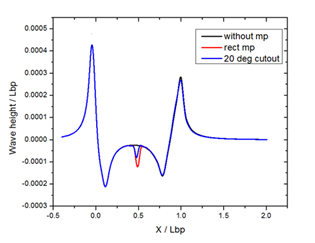Figure 4(C) Free surface elevation in 20 degree cut-out moonpool.Figure 4(D) Free surface elevation in 30 degree cut-out moonpool.

Pressure on the hull
The pressure distribution over the bow part of the drillship from XPAN at Froude number 0.0393 is given in Figure 5.The pressure distribution is given in the form of pressure coefficient, it can be find panel wise. This pressure distribution over the hull is used for the calculation of wave resistance. The total pressure acing along the hull is directly proportional to the wetted surface area, velocity and density of salt water. The pressure coefficient is simulated using panel method. The pressure distribution over the hull is used to find the critical regions. High fluctuation of pressure along the waterline represents the wave crest and the trough. The drillship have higher block coefficient like tanker vessel, the wave making resistance/wave breaking resistance have higher value near the forward shoulder part of the vessel. That can be found by pressure integration of over the hull.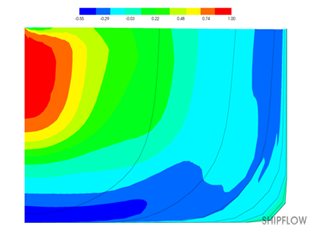Figure 5 Pressure distributions over the bulbous bow.

Free surface around the hull
The incompressible free surface flow around the drillship hull without moonpool and 20 degree cut-out moonpool at Froude number 0.0393 is given in Figure 6(A) & Figure 6(B) respectively. At lower Froude number the contours around the hull is small because of the small wave making resistance. The flow around the hull, with moonpool is disturbed in the moonpool region due to the main flow velocity of the flow is disturbed. This disturbance is shown in Figure 6(B).Figure 6(A) Free surface around a bare hull.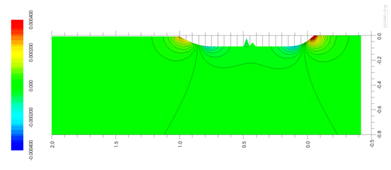Figure 6(B) Free surface around the drillship hull with 20 degree cut-out moonpool.

The free surface flow distribution around the hull shows the relationship between the Froude number and the flow pattern.

Total resistance
The total resistance coefficient (CT) from the simulation is the combination of wave resistance coefficient (Cw), viscous resistance coefficient (Cv). In the viscous part; frictional resistance coefficient (CF) and viscous pressure resistance coefficient (CPV) are counted. This viscous part is added with the wave resistance part and the total resistance coefficient is evaluated.
$\begin{array}{l}{C}_{v}={C}_{F}+{C}_{PV}\\ {C}_{T}={C}_{v}+{C}_{w}\\ {C}_{T}=\frac{{R}_{T}}{0.5\rho S{V}^{2}}\end{array}$

The total resistance (RT) of the vessels is directly proportional to the wetted surface area, speed of the vessel and density. The total resistance of the drillship against different Froude number is plotted in Figure 7.

For the validation of numerical calculation results, bare hull model towed in the towing tank for various Froude numbers as well as different loading conditions. The total resistance of bare hull from the experiment at maximum loaded draft condition has compared with the numerical calculation result as shown in Figure 7.

# Conclusion

The total resistance of the drillship and the effect of free surface with different moonpool configurations are discussed in this paper. The computer simulation conducted for different Froude numbers in the loaded draft of the drillship. The XCHAP simulation runs up to 5000 iterations for convergence results. The moonpool with cut-out angles are good in controlling the free surface elevation inside the moonpool as compared with the rectangular moonpool. In the case of resistance the moonpools are increase the resistance of ship when it is in forward speed condition. The moonpool with cut-out angle give the resistance in between the rectangular moonpool and hull without moonpool.

Nomenclature

$\varphi$ :          Flow potential

$\upsilon$ :          Kinematic viscosity (m-2 s-1)

$\rho$ :         Density of water (kg m-3)

P:            Pressure (N m-2)

Fn:           Froude number

V:            Wetted surface area (m-2)

S:            Wetted surface area (m-2)

$\mu$ :         Dynamic viscosity (N s m-2)

X:            Position along the length of the vessel

None.

# Conflict of interest

Author declares there is no conflict of interest in publishing the article.